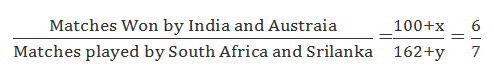# High Level Missing Data Interpretation For IBPS PO Set-16Things to keep in mind before attempting Missing DI Questions for IBPS PO-7
• Missing DI questions are another form of Tabular DI with some data missing.
• Mostly you will be asked to find the missing data, by giving some condition to follow while solving.
• In some cases, questions may be indirect, but still, there will be a link between the known data and given a condition.
• Once you identified the link, it will be easy for you to solve the question quickly.
• After finding the link, if you feel it is a lengthy process and it is going to consume more time to solve, it is better to skip it and mark it for review and solve it at the end if time permits.
• Some are scared to attempt missing DI, but the real fact is it is one of the easiest types of the question if you can able to find the link and approach the question smartly.
• Usually out of 5 questions, at least 2 will be easy, 1 or 2 will be lengthy and 1 or 2 will be lengthy as well as difficult.
• So it is better to identify the difficulty level of the question, by reading it properly before solving them.
• To identify the difficulty level of a particular question easily, the only thing you have to do is Practice, Practice and Practice a lot of Missing DI set before your exam.
Let us discuss a typical type of Missing DI set with a detailed solution:

(Direction 1 -5) Following is a tabulation of Number of ODI Cricket Matches Played, won, lost and drawn by five different countries in the year 2016 with some missing data. Read the table carefully and answer the questions.

Country
Name
Total No. of Matches Played No. of Matches Won No. of Matches Lost No. of Matches Draw
Australia
-
-
43
12
India
-
100
44
-
Pakistan
150
90
-
-
South Africa
162
-
47
-
Sri Lanka
-
80
-
-

### Question 1.

How many countries has won more than 100 matches, if the total number of matches won by all the country is 475 and total number of matches played by Australia is twice as match won by Srilanka?
A. None
B. One
C. Two
D. Three
E. None of these

### Question 2.

What is the number of matches draw by Srilanka, if the ratio between matches won by India and Australia to the number of matches played by South Africa and Srilanka is 6:7 and the ratio between number of matches played by Australia and Pakistan to number of matches lost by South Africa and Srilanka is 3:1, given that number of matches lost by Srilanka is twice the number of matches lost by India?
A. 15
B. 18
C. 20
D. Data Insufficient
E. None of these

### Question 3.

What is the total number of matches played by India, if the number of matches draw by India is 10% of the matches won by India?
A.154
B. 155
C. 164
D. 165
E. None of these

### Question 4.

If Pakistan didn’t lose any match, then what is the difference between the percentage of matches draw by Pakistan and percentage of matches lost by South Africa?
A. 15
B. 16
C. 17
D. Data Insufficient
E. None of these

### Question 5.

If none of the match was draw by any of the country, then what will be the ratio between matches played by India, Pakistan and South Africa to number of matches lost by India, Pakistan and Australia?
A.155:49
B.155:52
C.153: 49
D.152:49
E. None of these

### 1. Option B

By observing the table, we can predict the answer as either One or Two.
Total no. of matches played by Australia = 2 * match won by Srilanka
= 2*80 = 160
No. of matches won by Australia = 160- (43+12) =105 (> 100)
Total no. of matches won by South Africa= 475- (105+100+90+80)
= 100 (not greater than 100)
Only Australia has won more than 100 matches, so the answer is one.

### 2. Option C

Let matches won by Australia be x
Let matches played by Srilanka be y7(100 +x) = 6(162+y)
700 + 7x = 972 + 6y
7x – 6y = 972 – 700
7x – 6y = 272 -----------------(1)
Let the matches played by Australia be z
Matches lost by Srilanka = 2*44=88z+150= 3(135)
z = 405 – 150 = 255 (matches played by Australia)
Matches won by Australia (x) = z – (43 + 12) = 255-(43+12) =200
Sub x= 200 in eqn. (1)
7(200) – 6y = 272
1400 -6y = 272
6y= 1128
Y = 188 (matches played by Srilanka)
Therefore, matches draw by Srilanka= 188- (80 +88) =20 (ans)

### 3. Option A

No. of matches draw by India = (10/100)* 100 = 10
No. of matches Played by India =100 + 44 +10 = 154 (ans)

### 4. Option E

No. of matches draw by Pakistan= 150- (90+0) = 60
% of matches draw by Pakistan = (60/150)* 100 =40 %
% of matches lost by South Africa = (47/162) * 100 = 29 %
Difference = 40 – 29 = 11(ans)

### 5. Option D

Matches played by India =100+44 =144
Matches played by Pakistan = 150
Matches played by South Africa = 162
Matches lost by India =44
Matches lost by Pakistan = 150 – 90 = 60
Matches lost by Australia = 43#### What's trending in BankExamsToday

Smart Prep Kit for Banking Exams by Ramandeep Singh - Download here